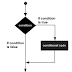R as a programming language was developed by Ross Ihaka and Robert Gentleman in 1993. It is a  language and environment for statistical computing and graphics.

2. Now you need RStudio, which is an application that helps you write in R . So, for this again do a  search for the latest version and install it on your computer using default settings.

• Select the free version RStudio Desktop.
• Select the OS of your device on which you are going to install. The recommendation would show if not select one.
• You would get the RStudio-version.exe file downloaded. (Ex. RStudio-1.4.1717.exe in Windows)
• Run the file to install. Click 'Yes' when it asks for permission to install and follow the steps accordingly and wait until you get your device installed with RStudio.

3. Once you download both of the applications, you can then begin using R by opening the Rstudio program.

#### R packages

Before working on R in R studio, you must install the R packages. The easiest way to install an R package is to use the command.

install.packages ("<package_name>")

### R- Data Types

In R, variables are assigned with R-objects and the data type of the R-object is the data type of the variables. The frequently used R-objects are Vectors, Lists, Matrices, Arrays, Factors, and Data frames.

#### 1.Vector

The simplest object is the vector object and there are six data types of these atomic vectors. In creating a vector with more than one element, you should c() function which means to combine the elements into a vector.

#### 2. Lists

A list is an R-object that can contain many different types of elements inside it like vectors, functions, and even another list inside it.

#### 3. Matrix

A matrix is a two-dimensional rectangular dataset. It can be created using a vector input to the matrix function.

#### 4. Factors

Factors are r-objects that are created using a vector. It stores the vectors with the distinct values of the elements in the vector as labels.

#### 5. Data frames

Data frames are tabular data objects. Unlike a matrix in a data frame, each column can contain different modes of data. This is a list of vectors of equal length. Data frames are created using the data.frame() function.

### R Operators

An operator is a symbol that tells the compiler to perform specific mathematical or logical manipulations. Following types of operators in R Programming are used.
• Arithmetic operators
• Relational operators
• Logical operators
• Assignment Operators
• Miscellaneous operators

### R Decision Making

Decision making structures require the programmer to specify one or more conditions to be evaluated or tested by the program, along with a statement or statements to be executed if the condition is determined to be true, and optionally, other statements to be executed if the condition is determined to be false.

#### a. IF statement

Syntax

if (Boolean_expression) {
// statement(s) will execute if Boolean expression is true
}

#### b. IF ELSE statement

if (Boolean_expression) {
// statement(s) will execute if Boolean expression is true
} else {
// statement(s) will execute if Boolean expression is false
}

### R Loop types

#### a. Repeat loop

Executes a sequence of statements multiple times and abbreviates the code that manages the loop variable.

#### b. While loop

Repeats a statement or group of statements while a given condition is true. It tests the condition before executing the loop body.

#### c. For loop

Like a while statement, except that it tests the condition at the end of the loop body.

Getting started with R and BasicsReviewed by SAGAR on July 15, 2021 Rating: 5